# http www comp nus edu sgcs 1010 Unit

• Slides: 23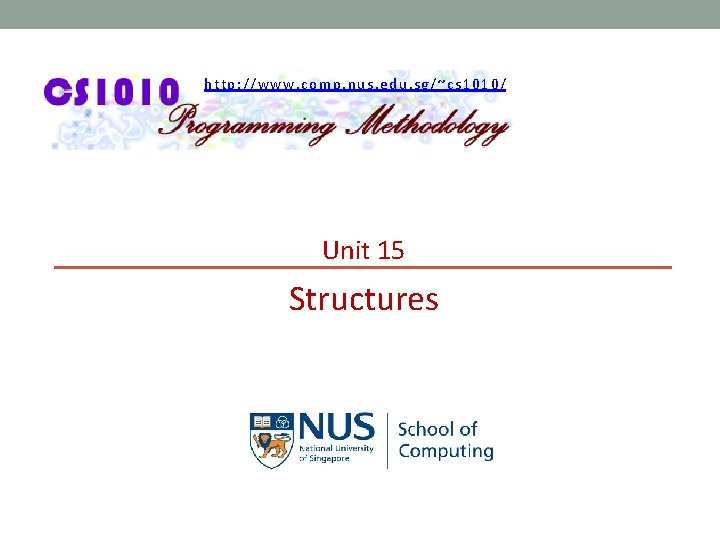http: //www. comp. nus. edu. sg/~cs 1010/ Unit 15 Structures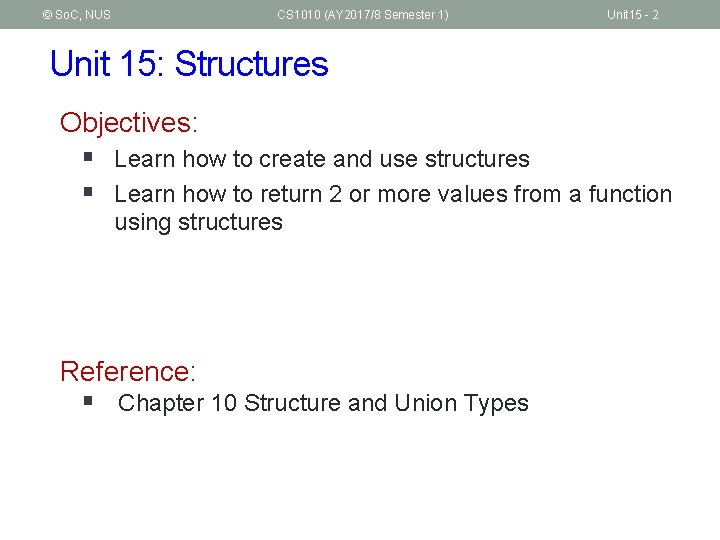© So. C, NUS CS 1010 (AY 2017/8 Semester 1) Unit 15 - 2 Unit 15: Structures Objectives: § Learn how to create and use structures § Learn how to return 2 or more values from a function using structures Reference: § Chapter 10 Structure and Union Types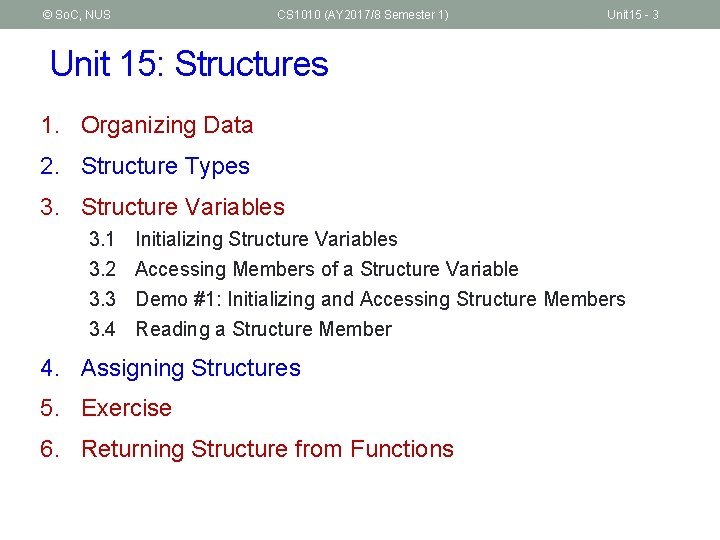© So. C, NUS CS 1010 (AY 2017/8 Semester 1) Unit 15 - 3 Unit 15: Structures 1. Organizing Data 2. Structure Types 3. Structure Variables 3. 1 3. 2 3. 3 3. 4 Initializing Structure Variables Accessing Members of a Structure Variable Demo #1: Initializing and Accessing Structure Members Reading a Structure Member 4. Assigning Structures 5. Exercise 6. Returning Structure from Functions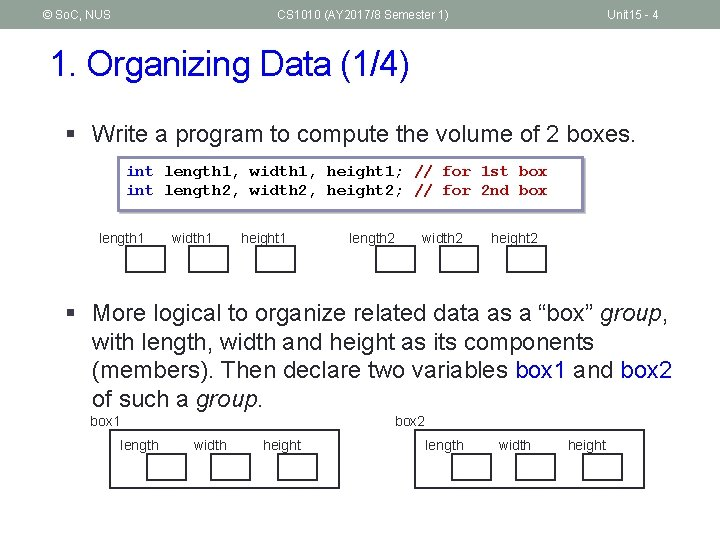© So. C, NUS CS 1010 (AY 2017/8 Semester 1) Unit 15 - 4 1. Organizing Data (1/4) § Write a program to compute the volume of 2 boxes. int length 1, width 1, height 1; // for 1 st box int length 2, width 2, height 2; // for 2 nd box length 1 width 1 height 1 length 2 width 2 height 2 § More logical to organize related data as a “box” group, with length, width and height as its components (members). Then declare two variables box 1 and box 2 of such a group. box 1 box 2 length width height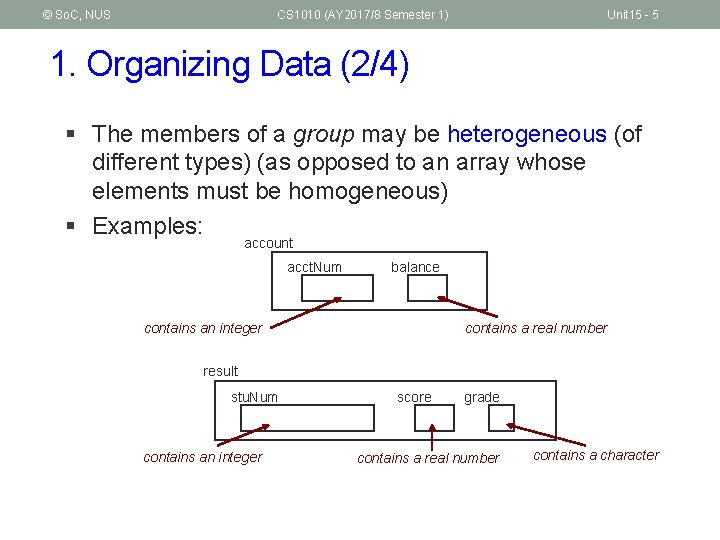© So. C, NUS CS 1010 (AY 2017/8 Semester 1) Unit 15 - 5 1. Organizing Data (2/4) § The members of a group may be heterogeneous (of different types) (as opposed to an array whose elements must be homogeneous) § Examples: account acct. Num balance contains an integer contains a real number result stu. Num contains an integer score grade contains a real number contains a character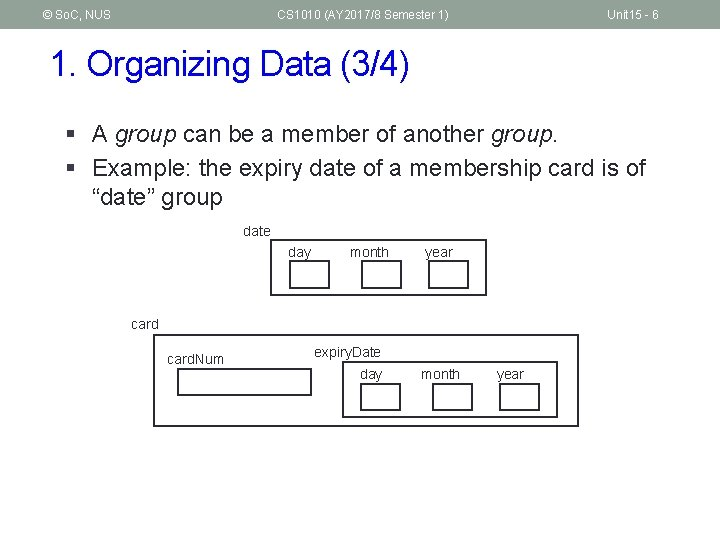© So. C, NUS CS 1010 (AY 2017/8 Semester 1) Unit 15 - 6 1. Organizing Data (3/4) § A group can be a member of another group. § Example: the expiry date of a membership card is of “date” group date day month year card. Num expiry. Date day month year© So. C, NUS CS 1010 (AY 2017/8 Semester 1) Unit 15 - 7 1. Organizing Data (4/4) § We can also create array of groups § Example: enrolment data for modules § Using two parallel arrays o codes[i] and enrolments[i] are related to the same module i § Using an array of “module” group § Which is more logical? § To be covered later in Unit 18 enrolments codes CS 1010 292 CS 1234 178 CS 1010 E 358 : : modules CS 1010 292 CS 1234 178 CS 1010 E 358 :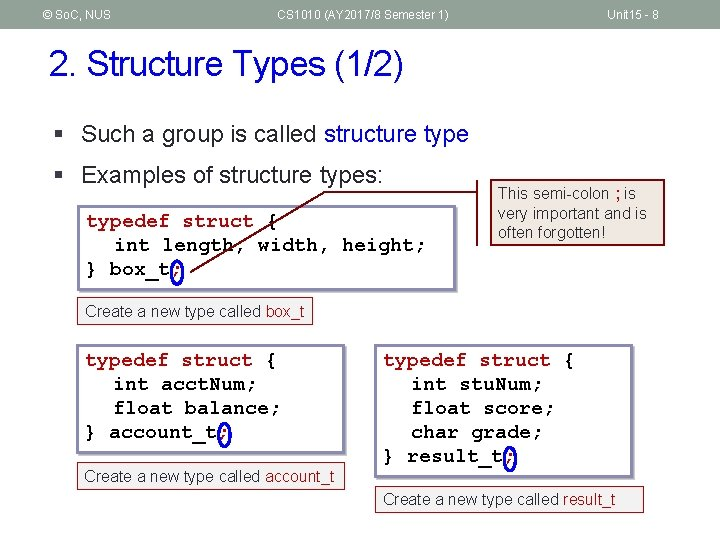© So. C, NUS CS 1010 (AY 2017/8 Semester 1) Unit 15 - 8 2. Structure Types (1/2) § Such a group is called structure type § Examples of structure types: typedef struct { int length, width, height; } box_t; This semi-colon ; is very important and is often forgotten! Create a new type called box_t typedef struct { int acct. Num; float balance; } account_t; Create a new type called account_t typedef struct { int stu. Num; float score; char grade; } result_t; Create a new type called result_t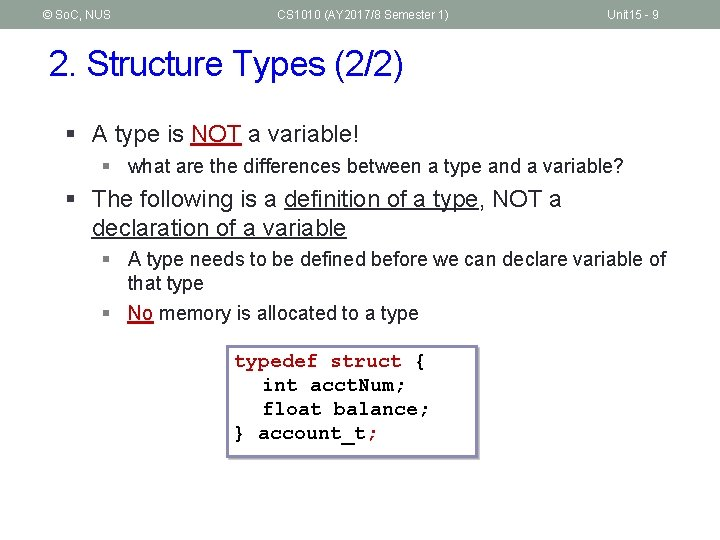© So. C, NUS CS 1010 (AY 2017/8 Semester 1) Unit 15 - 9 2. Structure Types (2/2) § A type is NOT a variable! § what are the differences between a type and a variable? § The following is a definition of a type, NOT a declaration of a variable § A type needs to be defined before we can declare variable of that type § No memory is allocated to a typedef struct { int acct. Num; float balance; } account_t;© So. C, NUS CS 1010 (AY 2017/8 Semester 1) 3. Structure Variables § Declaration § The syntax is similar to declaring ordinary variables. typedef struct { int stu. Num; float score; char grade; } result_t; Before function prototypes (but after preprocessor directives) result_t result 1, result 2; Inside any function Unit 15 - 10© So. C, NUS CS 1010 (AY 2017/8 Semester 1) Unit 15 - 11 3. 1 Initializing Structure Variables § The syntax is like array initialization § Examples: typedef struct { int day, month, year; } date_t; typedef struct { int stu. Num; float score; char grade; } result_t; typedef struct { int card. Num; date_t birthday; } card_t; card_t card 1 = {888888, {31, 12, 2020}}; result_t result 1 = { 123321, 93. 5, 'A' };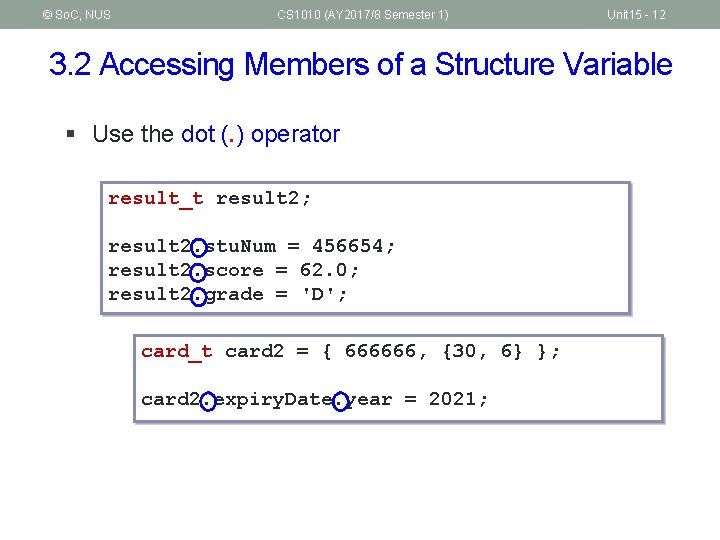© So. C, NUS CS 1010 (AY 2017/8 Semester 1) Unit 15 - 12 3. 2 Accessing Members of a Structure Variable § Use the dot (. ) operator result_t result 2; result 2. stu. Num = 456654; result 2. score = 62. 0; result 2. grade = 'D'; card_t card 2 = { 666666, {30, 6} }; card 2. expiry. Date. year = 2021;© So. C, NUS CS 1010 (AY 2017/8 Semester 1) Unit 15 - 13 3. 3 Demo #1: Initializing and Accessing Members Unit 15_Demo 1. c #include <stdio. h> typedef struct { int stu. Num; float score; char grade; } result_t; result 1: stu. Num = 123321; score = 93. 5; grade = A result 2: stu. Num = 456654; score = 62. 0; grade = D Type definition int main(void) { result_t result 1 = { 123321, 93. 5, 'A' }, result 2; result 2. stu. Num = 456654; result 2. score = 62. 0; result 2. grade = 'D'; Accessing members printf("result 1: stu. Num = %d; score = result 1. stu. Num, result 1. score, printf("result 2: stu. Num = %d; score = result 2. stu. Num, result 2. score, return 0; } Initialization %. 1 f; grade = %cn", result 1. grade); %. 1 f; grade = %cn", result 2. grade);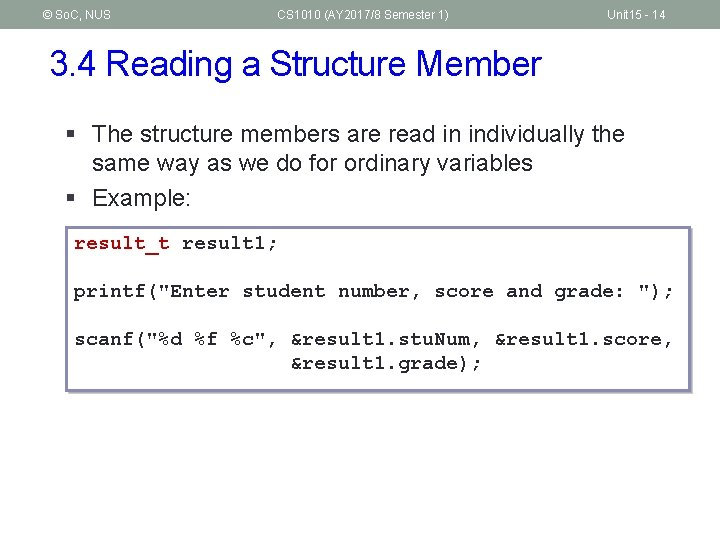© So. C, NUS CS 1010 (AY 2017/8 Semester 1) Unit 15 - 14 3. 4 Reading a Structure Member § The structure members are read in individually the same way as we do for ordinary variables § Example: result_t result 1; printf("Enter student number, score and grade: "); scanf("%d %f %c", &result 1. stu. Num, &result 1. score, &result 1. grade);© So. C, NUS CS 1010 (AY 2017/8 Semester 1) Unit 15 - 15 4. Assigning Structures § We use the dot operator (. ) to access individual member of a structure variable. § If we use the structure variable’s name, we are referring to the entire structure. § Unlike arrays, we may do assignments with structures result 2 = result 1; Before: result 1 stu. Num 123321 result 2 stu. Num 456654 = result 2. stu. Num = result 1. stu. Num; result 2. score = result 1. score; result 2. grade = result 1. grade; After: score 93. 5 score 62. 0 grade 'A' grade 'D' result 1 stu. Num 123321 result 2 stu. Num 123321 score 93. 5 grade 'A'© So. C, NUS CS 1010 (AY 2017/8 Semester 1) Week 8 - 16 5. Exercise #1: Perimeter (1/2) § Write a program Unit 15_Perimeter. c to do the following: 1. Define a structure type rectangle_t with 2 integer members: side 1 and side 2, which are the lengths of its 2 sides. 2. Declare a variable of type rectangle_t and read values into its members. 3. Compute the minimum perimeter if we fold the rectangle into halves once, either along the x-axis or the y-axis. § Note § Do not use any additional variables besides the two given variables. § You may write the code in the main() function. You may modularise the program later.© So. C, NUS CS 1010 (AY 2017/8 Semester 1) Week 8 - 17 5. Exercise #1: Perimeter (2/2) #include <stdio. h> Unit 15_Perimeter. c typedef struct { int side 1, side 2; } rectangle_t; int main(void) { rectangle_t rect; int perimeter; printf("Enter lengths: "); scanf("%d %d", &rect. side 1, &rect. side 2); if (rect. side 1 > rect. side 2) perimeter = rect. side 1 + 2*rect. side 2; else perimeter = rect. side 2 + 2*rect. side 1; printf("Perimeter = %dn", perimeter); return 0; }© So. C, NUS CS 1010 (AY 2017/8 Semester 1) Unit 15 - 18 6. Returning Structure from Functions (1/4) § When combined with arrays and functions, structures give us a lot of flexibility in organizing and passing around data. § One such example is that a function may return more than one outputs using structure. § We will explore other examples later in Unit #18.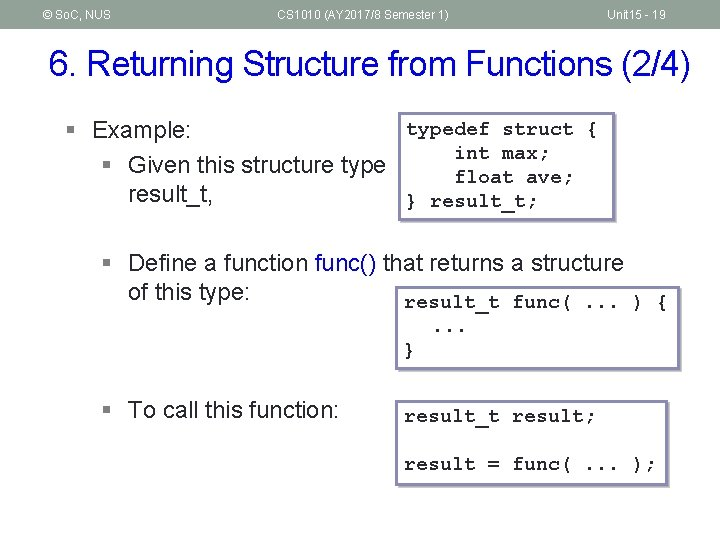© So. C, NUS CS 1010 (AY 2017/8 Semester 1) Unit 15 - 19 6. Returning Structure from Functions (2/4) typedef struct { § Example: int max; § Given this structure type float ave; result_t, } result_t; § Define a function func() that returns a structure of this type: result_t func(. . . ) {. . . } § To call this function: result_t result; result = func(. . . );© So. C, NUS CS 1010 (AY 2017/8 Semester 1) Unit 15 - 20 6. Returning Structure from Functions (3/4) #include <stdio. h> Unit 15_Demo 2. c typedef struct { int max; float ave; } result_t; result_t max_and_average(int, int); int main(void) { int num 1, num 2, num 3; // inputs result_t result; returned structure is printf("Enter 3 integers: "); copied to result scanf("%d %d %d", &num 1, &num 2, &num 3); result = max_and_average(num 1, num 2, num 3); }. . . printf("Maximum = %dn", result. max); printf("Average = %. 2 fn", result. ave); max and average return 0; are printed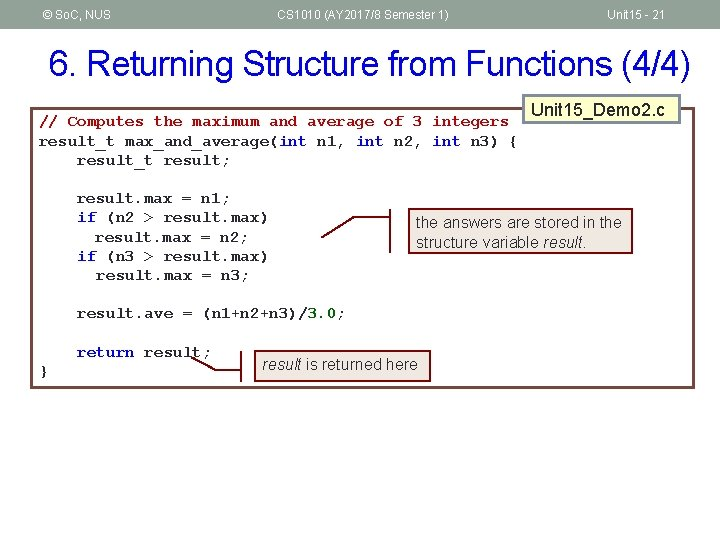© So. C, NUS CS 1010 (AY 2017/8 Semester 1) Unit 15 - 21 6. Returning Structure from Functions (4/4) // Computes the maximum and average of 3 integers result_t max_and_average(int n 1, int n 2, int n 3) { result_t result; result. max = n 1; if (n 2 > result. max) result. max = n 2; if (n 3 > result. max) result. max = n 3; the answers are stored in the structure variable result. ave = (n 1+n 2+n 3)/3. 0; return result; } Unit 15_Demo 2. c result is returned here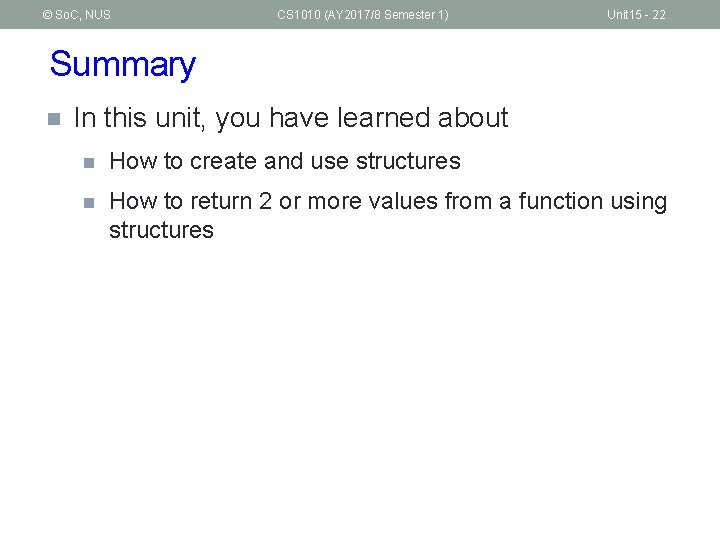© So. C, NUS CS 1010 (AY 2017/8 Semester 1) Unit 15 - 22 Summary n In this unit, you have learned about n How to create and use structures n How to return 2 or more values from a function using structures© So. C, NUS CS 1010 (AY 2017/8 Semester 1) End of File Unit 15 - 23